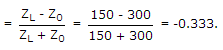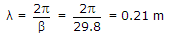# Electronics and Communication Engineering - Microwave Communication

### Exercise :: Microwave Communication - Section 1

26.

A line has Z0 = 300 ∠ 0° Ω. If ZL = 150 ∠ 0° Ω, reflection coefficient is

 A. 0.5 B. 0.3333 C. -0.3333 D. -0.5

Explanation:

Reflection coefficient27.

Assertion (A): A backward wave oscillator has an internal positive feedback.

Reason (R): A positive feedback is necessary for sustained oscillations.

 A. Both A and R are correct and R is correct explanation of A B. Both A and R are correct but R is not correct explanation of A C. A is correct but R is wrong D. A is wrong but R is correct

Explanation:

It is somewhat similar to TWT and can deliver microwave power over a wide frequency band.

It has an electron gun and a helix structure. However the interaction between electron beam and RF wave is different than in TWT.

The growing RF wave travels in opposite direction to the electron beam.

The frequency of wave can be changed by changing the voltage which controls the beam velocity.

Moreover the amplitude of oscillations can be decreased continuously to zero by changing the beam current.

It features are:

1. Frequency range - 1 GHz to 1000 GHz.

2. Power output - 10 mV to 150 mW (continuous wave) 250kW (pulsed).

It is used as signal source in transmitters and instruments.

28.

In a microstrip transmission the signal can be easily redirected by changing the centre strip.

 A. True B. False

Explanation:

A Microstrip line has a single dielectric substratc with ground plane on one side and a strip on the other face.

The high dielectric constant of the substrate reduces guide wavelength and circuit dimensions.

A microstrip line is the most commomly used transmission structure for microwave integrated circuits.

29.

The frequency of oscillation in a backward wave oscillator can be changed by

 A. varying the voltage which controls beam velocity B. varying the beam current C. both by varying the beam current and by light varying the voltage which controls beam velocity D. changing the rate of thermionic emission

Explanation:

It is somewhat similar to TWT and can deliver microwave power over a wide frequency band.

It has an electron gun and a helix structure. However the interaction between electron beam and RF wave is different than in TWT.

The growing RF wave travels in opposite direction to the electron beam.

The frequency of wave can be changed by changing the voltage which controls the beam velocity.

Moreover the amplitude of oscillations can be decreased continuously to zero by changing the beam current.

It features are:

1. Frequency range - 1 GHz to 1000 GHz.

2. Power output - 10 mV to 150 mW (continuous wave) 250kW (pulsed).

It is used as signal source in transmitters and instruments.

30.

A line has a phase constant of 29.8 rad/m. At 1000 MHz the wavelength is

 A. 29.8 m B. 2.98 m C. 2.1 m D. 0.21 m.